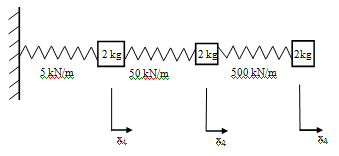## Calculate three natural frequencies of the system, MATLAB Programming

Assignment Help:

A three degree of freedom system is shown in Figure.  The three masses are each 1 kg and are constrained to move in the directions shown.   The three stiffnesses are   5 kN/m, 50 kN/m, and 500 kN/m as shown.

(a)  Use one and two degree of freedom approximations to estimate the three natural frequencies of the system in Hz.

(b)  Write the equations of motion of the system using matrices.

(c)  Using MATLAB or otherwise, calculate the three natural frequencies of the system and the associated mode shapes normalised on the largest value.

(d)  Find the principal stiffnesses for the system using the normalised mode shapes.#### Create matlab program, Create MATLAB program(s) that solve a problem of you...

Create MATLAB program(s) that solve a problem of your own choice. You may opt to solve work related problem, an industrial problem, or problem from your research project. Markin

#### Develop matlab monte carlo queuing simulator, Answers should be submitted i...

Answers should be submitted in an MS Word document. Simulations should accompany answers in a separate MATLAB file. 1)  Queuing Simulator: Consider a communications router that

#### Help with MATLAB, I have couple questions in MATLAB I need help in

I have couple questions in MATLAB I need help in

#### Wireless communication systems-matlab, Plot way forms for the following mod...

Plot way forms for the following modulation schemes using Matlab: a)      2 ASK                     b)  BFSK                                  c) BPSK          4 ASK

#### Math+Matlab, Read the document and quote me ... https://www.dropbox.com/s...

Read the document and quote me ... https://www.dropbox.com/s/pvq4f45fpwajibi/Course_wk%20_2013_.pdf

#### How to create a cell array, how to create a cell array

how to create a cell array

#### Plot the input and the output of the filter on a single plot, An FIR filter...

An FIR filter has coefficients b = [ 1.0000   -0.6387    1.0214    0.8210   -0.7470    1.0920 ] (a) Find H(z) for the filter and plot its frequency response (magnitude and phase

#### How to open simpower, How to Open Simpower: 1. Open MATLAB 2. ...

How to Open Simpower: 1. Open MATLAB 2. In the "Command Window" write ' simulink ' and press Enter or click on the Simulink icon. 3. Simulink Library Browser w

#### Script - matlab, Script - MATLAB: The script is a series of MATLAB ins...

Script - MATLAB: The script is a series of MATLAB instructions which is stored in a file and saved. The contents of a script can be showed in the Command Window by using the t

#### What are the matlab system parts, The MATLAB system having of five main par...

The MATLAB system having of five main parts: * Handle Graphics. * The MATLAB working environment. * The MATLAB language. * The MATLAB mathematical function library.